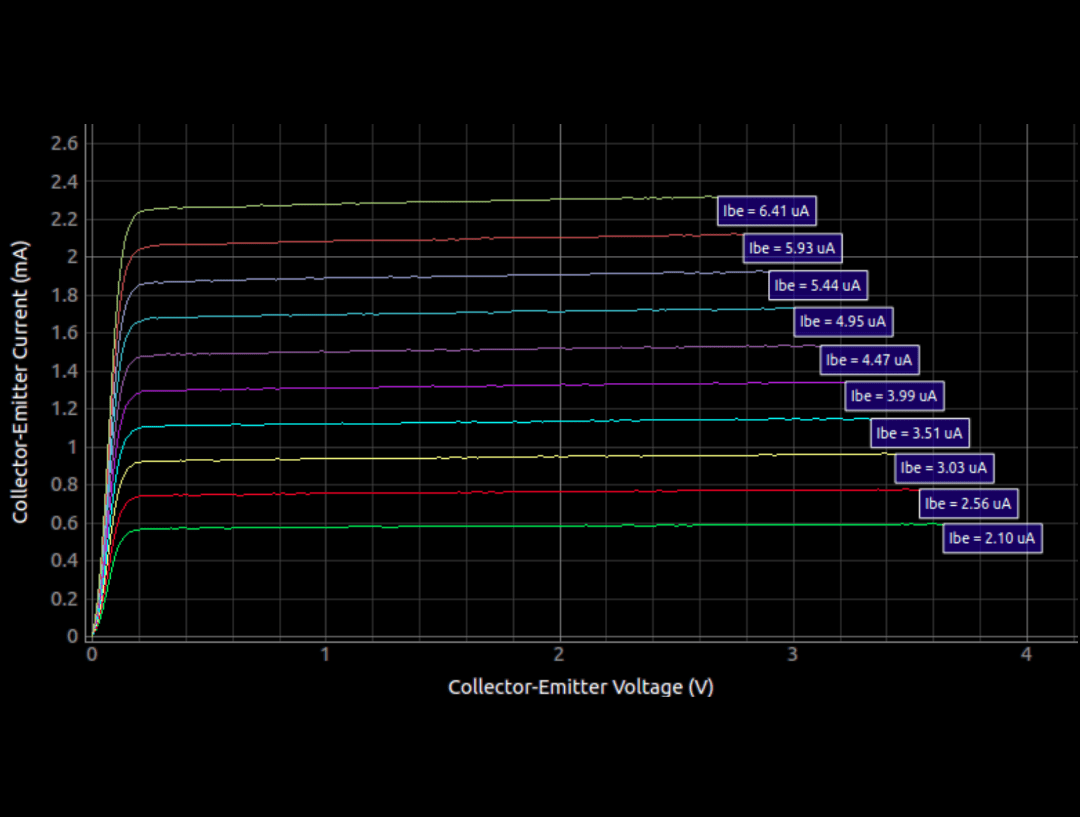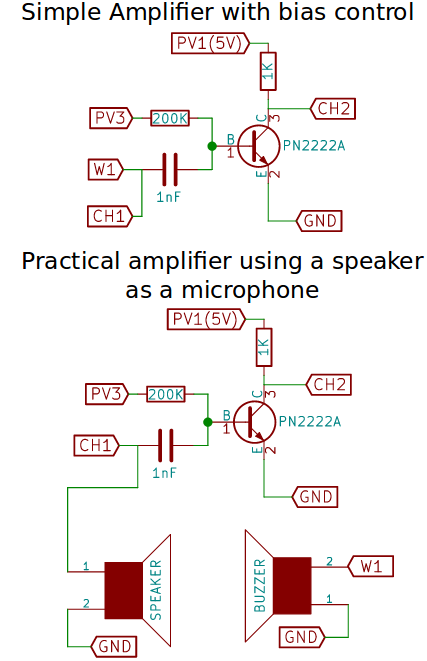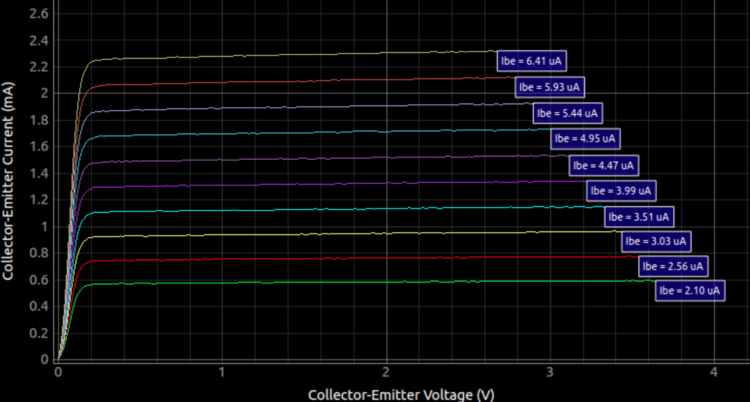Characteristization of Transistors Using PSLab

Transistors are one of the key building blocks of all electronics. They are fundamentally three-terminal semiconductor devices, with the terminals being labelled as the Emitter(E), Base(B), and Collector(C). These active components are found everywhere in electronics, and all of the complex processors that power everything from cellphones to aircraft employ millions of these devices in switching and amplification roles. In this blog post, we shall use the PSLab to explore some of the fundamental properties of transistors, and their various applications.

Transistor as an amplifierIn the schematic shown, we shall try to use an NPN transistor to amplify a small signal.

A small amplitude oscillation generated by W1 with the amplitude knob turned down to a very low level is used as the input. Since transistors do not handle bipolar signals, we have mixed a constant DC voltage generated PV3 to shift this small signal into the positive domain.

The fluctuating potential difference incident at the base of the transistor creates a corresponding current flow between the Base and Emitter.

By the fundamental property of transistors, this influences the path resistance between the Collector(C) and the Emitter(E) , and the resultant amplified voltage output can be monitored at the junction between the 1K resistor and the collector.

We have used CH1 to monitor the input voltage, and CH2 for the output

In a more applied scenario, we can implement the second schematic in order to create an audio amplifier. Instead of using W1 as the input signal, a speaker is used as a microphone. When a sound signal is incident on the speaker, its membrane oscillates, and as a result , the coil attached to it also does the same. Since this coil is placed in a magnetic field , its oscillations result in a change in the magnetic flux passing through, and this change causes a voltage(EMF) induced at its output. We then use our transistor amplifier to amplify this small EMFFigure 2 : A Transistor being used to apply a gain of 81.5x to a small amplitude sine wave. The input waveform (green) is shown on a +/-500mV full scale and the output waveform is shown on a +/-8V scale in order to be able to view both. However, due to the difference in scales, the actual difference in amplitudes is 16 times more than what is visible.
Common Emitter Characteristics

Any introductory course on transistors includes a diagram similar to the one shown , and a description about how for any base current, the collector current eventually saturates, and that this saturation level is proportional to the base current itself.

With the PSLab’s transistor CE characterization app, we can set up this experiment, and verify this for ourselves using an NPN transistor. The results shown were gathered using a 2N2222 transistor

In the schematic , the base current is determined by the voltage source PV2, and a high value series resistor of 200 KOhms . We use an analog input CH3 to monitor the voltage present at the Base of the transistor in order to calculate the total base current.

Base current = V/R = (PV2 – CH3) / 200e3

Now that we have set a particular base current, PV1 is used to sequentially increase the voltage across the collector and emitter of the transistor. A current limiting resistor of 1K Ohm is used, and CH1 is used to monitor the voltage drop across the transistor.

Collector current = V/R = (PV1 – CH1) / 1e3

Plotting the behaviour of the collector current with respect to the collector voltage gives us the familiar current voltage characteristics of a transistor.Figure 3: Common emitter characteristics of an NPN transistor (2N2222) for various base currents

We can now alter the base current by changing PV2, and verify that the saturation current for the collector is indeed a function of it.